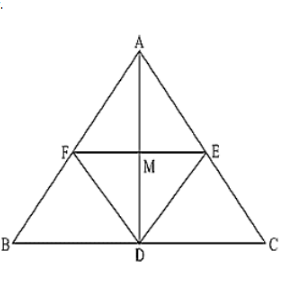# Let ABC be an isosceles triangle in which AB = AC. If D, E, F be the mid points of the, sides BC, CA and ABQuestion:

Let ABC be an isosceles triangle in which AB = AC. If D, E, F be the mid points of the, sides BC, CA and AB respectively, show that the segment AD and EF bisect each other at right angles.

Solution:

Since D, E and F are mid-points of sides BC, CA and AB respectively.

∴  AB ∥ DE and AC ∥ DF

∴  AF ∥ DE and AE ∥ DF

ABDE is a parallelogram.

AF = DE and AE = DF

(1/2) AB = DE and (1/2) AC = DF

DE = DF             [Since, AB = AC]

AE = AF = DE = DF

ABDF is a rhombus.

⟹ AD and FE bisect each other at right angle.